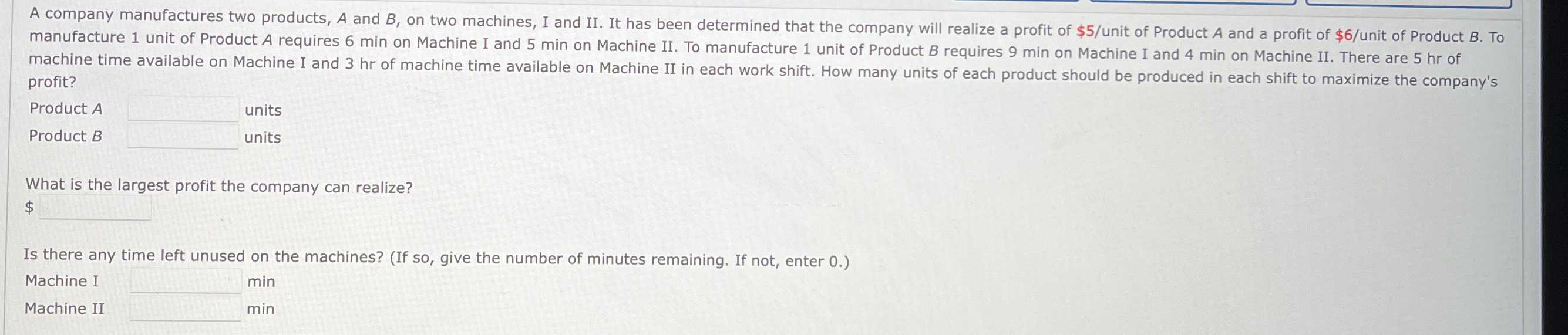### ¿Todavía tienes preguntas de matemáticas?

Pregunte a nuestros tutores expertos
Algebra
PreguntaA company manufactures two products, $$A$$ and $$B$$ , on two machines, I and II. It has been determined that the company will realize a profit of $$\ 5 /$$ unit of Product $$A$$ and a profit of $$\ 6 /$$ unit of Product $$B$$ . To manufacture $$1$$ unit of Product $$A$$ requires $$6 min$$ on Machine I and $$5 min$$ on Machine II. I. To manufacture $$1$$ unit of Product $$B$$ requires $$9 min$$ on Machine I and $$4 min$$ on Machine II. There are $$5 hr$$ of machine time available on Machine I and $$3 hr$$ of machine time available on Machine II in each work shift. How many units of each product should be produced in each shift to maximize the company's profit?

Product $$A$$ units

Product $$B$$ units

What is the largest profit the company can realize?

Is there any time left unused on the machines? (If so, give the number of minutes remaining. If not, enter $$0 .$$ )

Machine I_______ $$\min$$

Machine II_______ $$\min$$